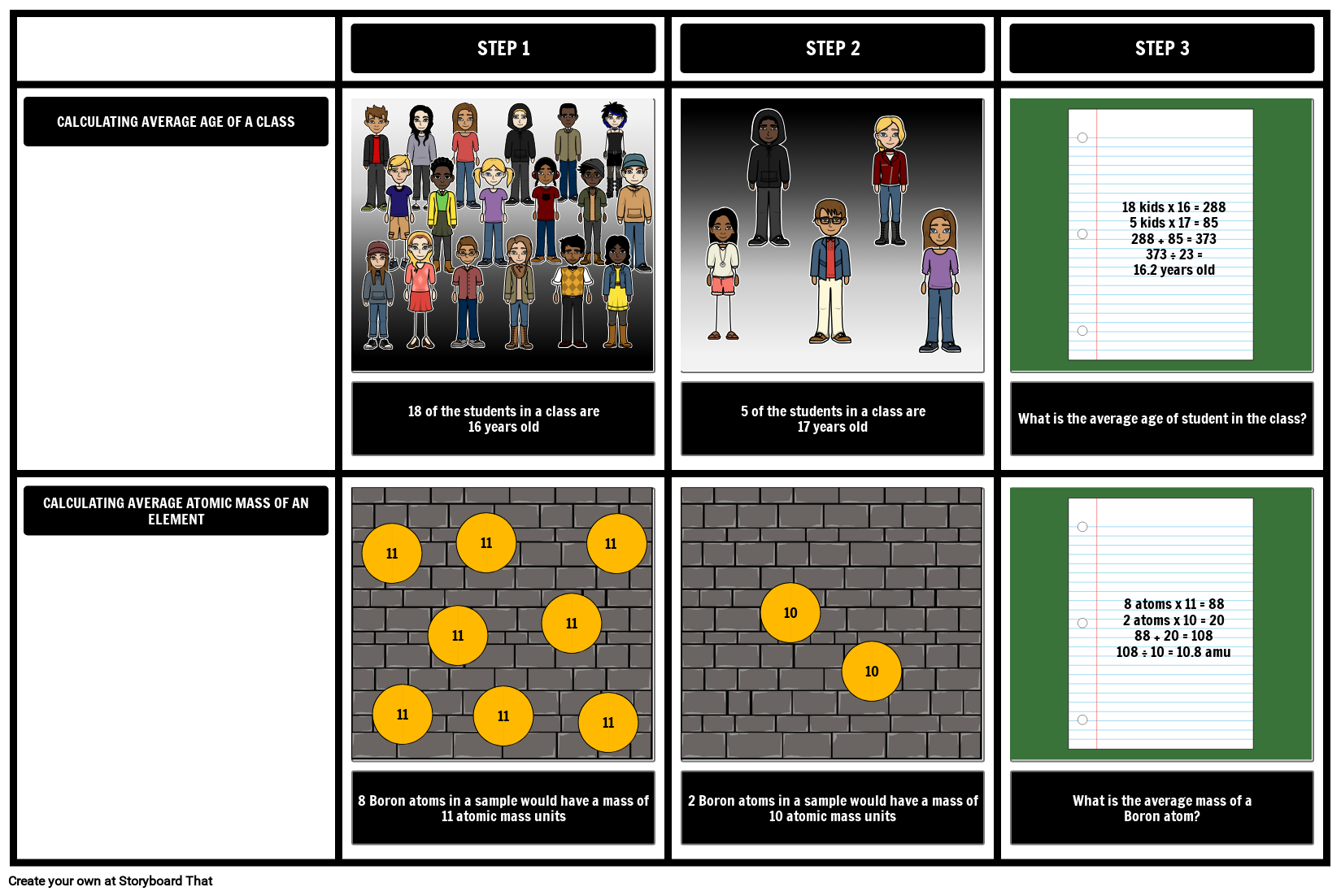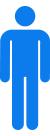What is Atomic Mass?## Activity Overview

Students will use the atomic mass of an element often throughout any chemistry course. One common misconception is that some atoms have a mass equal to the atomic mass, but that is false in many cases. Because the atomic mass is a weighted average that reflects the abundance of isotopes in nature, a model helps students better understand this concept.

In this activity, students will create examples to illustrate the percent abundances for an element and calculate the atomic mass for an element. This will help students understand this idea visually. The example above can also be edited and used as an example for students rather than an activity. Copying the assignment will also copy the completed example into your account!

In order to calculate the atomic mass of an element, scientists must know what isotopes exist and how abundant they are. Once this is known, the average atomic mass is calculated by taking into account the masses of the isotopes and how prevalent they are. This can be compared to finding the average age of students in a class. When most of the students are 16, but some of the students are 17, the average age can be predicted to be close to 16. Students typically understand this intuitively. The average age is not necessarily 16.5 (the average of 16 and 17) unless their are equal numbers of students who are 16 or 17. This model can be extended to isotopes: the more abundant an isotope, the closer the average will be to the mass of that isotope and the average will not necessarily be the midpoint between the masses of the isotopes.

Lesson Plan Reference

Difficulty Level 3 (Developing to Mastery)

Type of Assignment Individual or Partner

## Template and Class Instructions

(These instructions are completely customizable. After clicking "Use This Assignment With My Students", update the instructions on the Edit Tab of the assignment.)

#### Student Instructions

Create a chart that illustrates how to find the atomic mass of elements. Remember, the atomic mass is a weighted average.

1. Click "Start Assignment".
2. In the top row, calculate the average age of students in a class.
3. In the bottom row, do the same for the average atomic mass for an element.
4. Create an illustration to help visualize your calculations.
5. Save and submit your storyboard.
1x3 Grid Template## Education Pricing

This pricing structure is only available to academic institutions. Storyboard That accepts purchase orders.#### Single Teacher

As low as /month#### Department

As low as /month#### School/District

As low as /month

*(This will start a 2-Week Free Trial - No Credit Card Needed)
##### Over 15 Million Storyboards Created